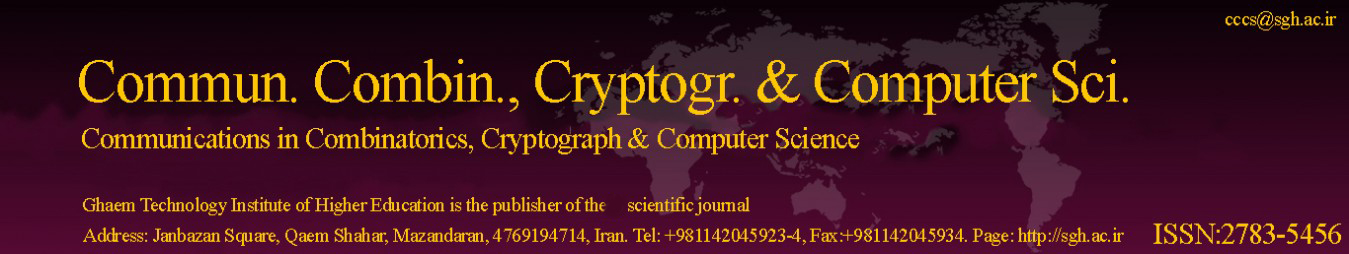Copy the following BibTeX for the article entitled Mostar Index of Conical and Generalized Gear Graph.

BibTeX

@article{Article_22,

title = {Mostar Index of Conical and Generalized Gear Graph},

journal = {Communications in Combinatorics, Cryptography & Computer Science},

volume = {2021},

issue = {2},

issn = { 2783-5456 },

year = {2021},

url = {http://cccs.sgh.ac.ir/Articles/2021/issue 2/2-10-_mostar_index_of_conical_and_generalized_gear_graph-1638859271.pdf},

author = {P. Kandan and S. Subramanian},

keywords = {Conical graph, Gear graph, Mostar index.},

abstract = {In theoretical chemistry, topological index play a significant role. Bond-additive index have been utilized more extensively than other topological indices that quantify graph peripherality. In this study, we compute the exact formula of one of the recently introduced bond-additive topological index called Mostar Index to the conical graph C(ℓ, k). Using the result obtained here, we have corrected the result obtained by Colako ˇ glu Havare. Moreover we obtained the Mostar index to the new graph called generalized gear graph C ∗ (ℓ, 2k).}

};